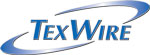###Nomenclature for NEMA HP3

Go Back

example number HP3- EXBGE9

HP3 - EX BG E9
|| || ||
Nema TypeConstruction Conductor Material AWG SizeStranding Color Code
E                 *
EE             **
EEE          ***
W = Wrapped
X = Extruded

B=Silver-coated Copper (SCC)
C=Nickel-coated Copper (NCC)
D=Silver-coated high-strength copper alloy (SCA)
E=Nickel-coated high-strength copper alloy (NCA)

A =32A =10 =BLK
B= 30B= 71= BRN
C=28 E=19 2=RED
D= 26G= 373= ORG
E= 24L= 1334= YEL

RECOMMENDED USES OF WIRE TYPES

F=22 P=665 5=GRN
G= 20R= 8176= BLU

* TYPE E

Type E is intended for high temperature applications and is considered suitable for use at a maximum of 600 volts RMS (root mean square) and/or high frequency circuitry.

H=18 S=1045 7=VLT
J=16 T=1330 8=GRY
K=14 V=1672 9=WHT
L= 12W= 2109

** TYPE EE

Type EE is intended for high temperature applications and is considered suitable for use at a maximum of 1000 volts RMS and/or for high frequency circuitry.

M =10
N=8
P=6
R= 4
*** TYPE ET

Type ET wire is intended for use in low voltage, high temperature applications.  It is considered suitable for use up to a maximum of 250 volts RMS and/or for high frequency circuitry.  Wires having ET thin wall insulation are relatively fragile and can be damaged.  They should not be used where mechanical stress or an abrasive environment may exist.

S=2
T=1
U=1/0
W=2/0
Y=3/0
Z =4/0

www.texwire.us   800-645-0073